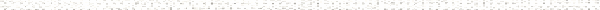A Precalculus Outline

 Chapter 9:  Sequences and the Binomial TheoremObjective: Introduction to SequencesObjective: Finding a formula for a sequenceObjective: What is an arithmetic sequence?Objective: What is a geometric sequence?Objective: Finding the nth term in an arithmetic sequenceObjective: Finding the nth term of a geometric sequenceObjective: Application of Arithmetic SequenceObjective: How to perform operations with factorialsObjective: Function notationObjective: Writing out sums expressed in summation (sigma) notation and writing sums in summation (sigma) notationObjective: Finding the partial sum of an arithmetic seriesObjective: Finding the partial sum of a geometric seriesObjective: Developing the formula for a mortgage payment using geometric seriesObjective: Using a Geometric Series to write a repeating decimal as a fractionObjective: Finding the sum of an (infinite) geometric seriesObjective: Introduction to SequencesObjective: Finding a formula for a sequenceObjective: What is an arithmetic sequence?Objective: What is a geometric sequence?Objective: Finding the nth term in an arithmetic sequenceObjective: Finding the nth term of a geometric sequenceObjective: Application of Arithmetic SequenceObjective: How to perform operations with factorialsObjective: Function notationObjective: Example proofs by inductionObjective: Using the Binomial Theorem to expand a binomialObjective: How to construct Pascal's TriangleObjective: Using Pascal's Triangle to expand a binomialObjective: The Binomial TheoremObjective: How to perform operations with factorialsObjective: Principle of Mathematical Induction